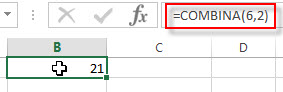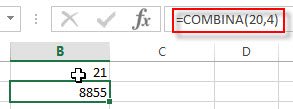## Excel COMBINA Function

This post will guide you how to use Excel COMBINA function with syntax and examples in Microsoft excel.

### Description

The Excel COMBINA function returns the number of combinations for a given number of items and it includes repetitions. So you can use the COMBINA function to calculate the number of combinations for a specified number of items, with repetitions. And this function will return a numeric value.

For example, assuming that you have 6 objects(a,b,c,d,e,f) and you can get 21 different combinations of 2 objects (with repetitions), the combinations are as follows:

`aa ab ac ad ae af bb bc bd be bf cc cd ce cf dd de df ee ef ff`

The COMBINA function is a build-in function in Microsoft Excel and it is categorized as a Math and Trigonometry Function.

The COMBINA function is available in Excel 2016, Excel 2013. And this function was introduced with Excel 2013.

### Syntax

The syntax of the COMBINA function is as below:

`=COMBINA(number, number_chosen)`

Where the COMBINAfunction arguments are:

• number –This is a required argument. Number is the total number of objects in the set. It must be greater than or equalt to 0, and it also must be greater than or equal to number_chosen argument.
• number_chosen – This is a required argument. The number of combinations that you want to. It must be greater than or equal to 0. If the value is not an integer, it will be truncated.

Note:

• If either the number argument or the number_chosen argument is less than 0, then the COMBINA function will return the #NUM! Error.
• If any arguments are non-numeric, this function will return #VALUE! Error.

### Excel COMBINA Function Examples

The below examples will show you how to use Excel COMBINA Function to get the number of combinations with repetitions for a given number objects from a set.

1# to get the number of combinations for 6 and 2(with repetitions), enter the following formula in Cell B1.

=COMBINA(6,2)2# to get the number of combinations for 20 and 4(with repetitions), enter the following formula in Cell B2.

=COMBINA(20,4)### Related Functions

• Excel COMBIN Function
The Excel COMBIN function returns the number of combinations for a given number of items. The syntax of the COMBIN function is as below:=COMBIN (number, number_chosen)…